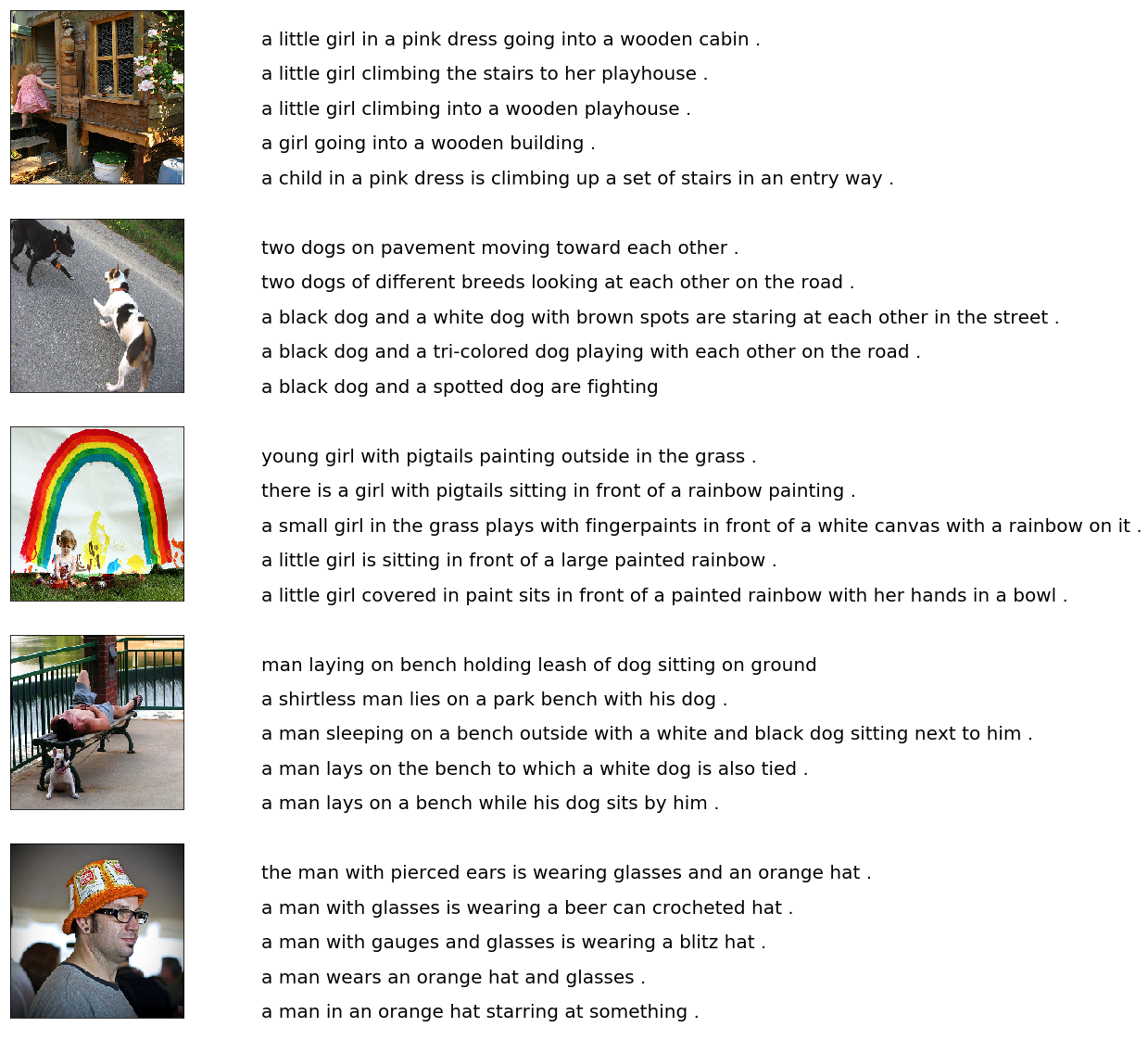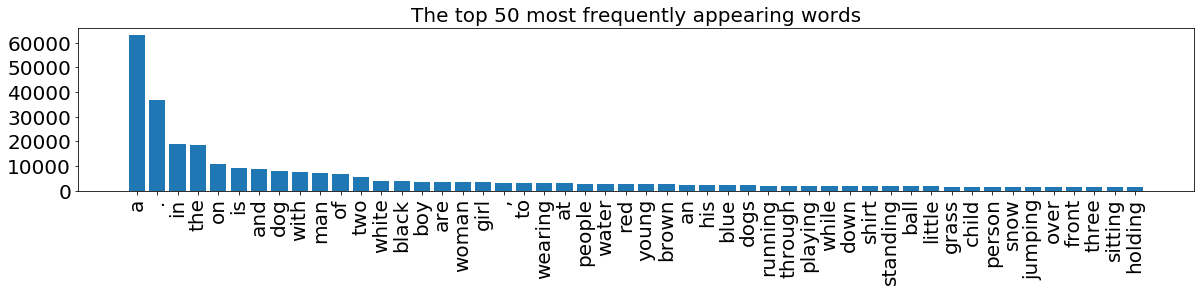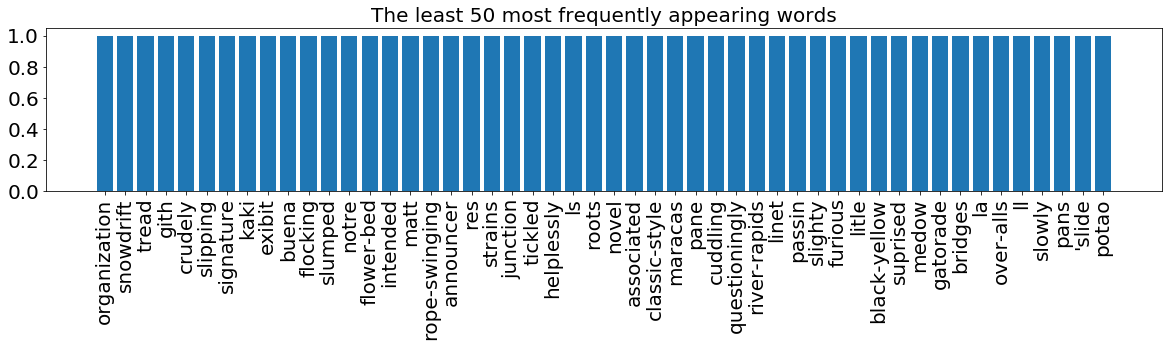# Develop an image captioning deep learning model using Flickr 8K data

Image captioning is an interesting problem, where you can learn both computer vision techniques and natural language processing techniques. In this blog post, I will follow How to Develop a Deep Learning Photo Caption Generator from Scratch and create an image caption generation model using Flicker 8K data. This model takes a single image as input and output the caption to this image.

To evaluate the model performance, I will use bilingual evaluation understudy BLEU. For this purpose, I will review the calculation of BLEU by going through its calculation step by step.

## Reference¶

How to Develop a Deep Learning Photo Caption Generator from Scratch

In :
import matplotlib.pyplot as plt
import tensorflow as tf
from keras.backend.tensorflow_backend import set_session
import keras
import sys, time, os, warnings
import numpy as np
import pandas as pd
from collections import Counter
warnings.filterwarnings("ignore")
print("python {}".format(sys.version))
print("keras version {}".format(keras.__version__)); del keras
print("tensorflow version {}".format(tf.__version__))
config = tf.ConfigProto()
config.gpu_options.per_process_gpu_memory_fraction = 0.95
config.gpu_options.visible_device_list = "0"
set_session(tf.Session(config=config))

def set_seed(sd=123):
from numpy.random import seed
from tensorflow import set_random_seed
import random as rn
## numpy random seed
seed(sd)
## core python's random number
rn.seed(sd)
## tensor flow's random number
set_random_seed(sd)

Using TensorFlow backend.

python 2.7.13 |Anaconda 4.3.1 (64-bit)| (default, Dec 20 2016, 23:09:15)
[GCC 4.4.7 20120313 (Red Hat 4.4.7-1)]
keras version 2.1.3
tensorflow version 1.5.0


Flilckr8K contains 8,000 images that are each paired with five different captions which provide clear descriptions of the salient entities and events. The images were chosen from six different Flickr groups, and tend not to contain any well-known people or locations, but were manually selected to depict a variety of scenes and situations.

In :
## The location of the Flickr8K_ photos
dir_Flickr_jpg = "../Flickr8k/Flicker8k_Dataset/"
## The location of the caption file
dir_Flickr_text = "../Flickr8k/Flickr8k.token.txt"

jpgs = os.listdir(dir_Flickr_jpg)
print("The number of jpg flies in Flicker8k: {}".format(len(jpgs)))

The number of jpg flies in Flicker8k: 8091


# Preliminary analysis¶

#### Import caption data¶

Load the text data and save it into a panda dataframe df_txt.

• filename : jpg file name
• index : unique ID for each caption for the same image
• caption : string of caption, all in lower case
In :
## read in the Flickr caption data
file = open(dir_Flickr_text,'r')
file.close()

datatxt = []
for line in text.split('\n'):
col = line.split('\t')
if len(col) == 1:
continue
w = col.split("#")
datatxt.append(w + [col.lower()])

df_txt = pd.DataFrame(datatxt,columns=["filename","index","caption"])

uni_filenames = np.unique(df_txt.filename.values)
print("The number of unique file names : {}".format(len(uni_filenames)))
print("The distribution of the number of captions for each image:")
Counter(Counter(df_txt.filename.values).values())

The number of unique file names : 8092
The distribution of the number of captions for each image:

Out:
Counter({5: 8092})

Let's have a look at some of the pictures together with the captions.

The 5 captions for each image share many common words and very similar meaning. Some sentences finish with "." but not all.

In :
from keras.preprocessing.image import load_img, img_to_array

npic = 5
npix = 224
target_size = (npix,npix,3)

count = 1
fig = plt.figure(figsize=(10,20))
for jpgfnm in uni_filenames[:npic]:
filename = dir_Flickr_jpg + '/' + jpgfnm
captions = list(df_txt["caption"].loc[df_txt["filename"]==jpgfnm].values)

count += 1

plt.axis('off')
ax.plot()
ax.set_xlim(0,1)
ax.set_ylim(0,len(captions))
for i, caption in enumerate(captions):
ax.text(0,i,caption,fontsize=20)
count += 1
plt.show()# Data preparation¶

We prepare text and image data separately.

## Text preparation¶

We create a new dataframe dfword to visualize distribution of the words. It contains each word and its frequency in the entire tokens in decreasing order.

In :
def df_word(df_txt):
vocabulary = []
for txt in df_txt.caption.values:
vocabulary.extend(txt.split())
print('Vocabulary Size: %d' % len(set(vocabulary)))
ct = Counter(vocabulary)
dfword = pd.DataFrame({"word":ct.keys(),"count":ct.values()})
dfword = dfword.sort("count",ascending=False)
dfword = dfword.reset_index()[["word","count"]]
return(dfword)
dfword = df_word(df_txt)

Vocabulary Size: 8918

Out:
word count
0 a 62989
1 . 36581
2 in 18975

### The most and least frequently appearing words¶

The most common words are articles such as "a", or "the", or punctuations.

These words do not have much infomation about the data.

In :
topn = 50

def plthist(dfsub, title="The top 50 most frequently appearing words"):
plt.figure(figsize=(20,3))
plt.bar(dfsub.index,dfsub["count"])
plt.yticks(fontsize=20)
plt.xticks(dfsub.index,dfsub["word"],rotation=90,fontsize=20)
plt.title(title,fontsize=20)
plt.show()

plthist(dfword.iloc[:topn,:],
title="The top 50 most frequently appearing words")
plthist(dfword.iloc[-topn:,:],
title="The least 50 most frequently appearing words")In order to clean the caption, I will create three functions that:

• remove punctuation
• remove single character
• remove numeric characters

To see how these functions work, I will process a single example string using these three functions.

In :
import string
text_original = "I ate 1000 apples and a banana. I have python v2.7. It's 2:30 pm. Could you buy me iphone7?"

print(text_original)
print("\nRemove punctuations..")
def remove_punctuation(text_original):
text_no_punctuation = text_original.translate(None, string.punctuation)
return(text_no_punctuation)
text_no_punctuation = remove_punctuation(text_original)
print(text_no_punctuation)

print("\nRemove a single character word..")
def remove_single_character(text):
text_len_more_than1 = ""
for word in text.split():
if len(word) > 1:
text_len_more_than1 += " " + word
return(text_len_more_than1)
text_len_more_than1 = remove_single_character(text_no_punctuation)
print(text_len_more_than1)

print("\nRemove words with numeric values..")
def remove_numeric(text,printTF=False):
text_no_numeric = ""
for word in text.split():
isalpha = word.isalpha()
if printTF:
print("    {:10} : {:}".format(word,isalpha))
if isalpha:
text_no_numeric += " " + word
return(text_no_numeric)
text_no_numeric = remove_numeric(text_len_more_than1,printTF=True)
print(text_no_numeric)

I ate 1000 apples and a banana. I have python v2.7. It's 2:30 pm. Could you buy me iphone7?

Remove punctuations..
I ate 1000 apples and a banana I have python v27 Its 230 pm Could you buy me iphone7

Remove a single character word..
ate 1000 apples and banana have python v27 Its 230 pm Could you buy me iphone7

Remove words with numeric values..
ate        : True
1000       : False
apples     : True
and        : True
banana     : True
have       : True
python     : True
v27        : False
Its        : True
230        : False
pm         : True
Could      : True
you        : True
me         : True
iphone7    : False
ate apples and banana have python Its pm Could you buy me


### Clean all captions¶

Using the three functions, I will clean all captions.

In :
def text_clean(text_original):
text = remove_punctuation(text_original)
text = remove_single_character(text)
text = remove_numeric(text)
return(text)

for i, caption in enumerate(df_txt.caption.values):
newcaption = text_clean(caption)
df_txt["caption"].iloc[i] = newcaption


After cleaning, the vocabularly size get reduced by about 200.

In :
dfword = df_word(df_txt)
plthist(dfword.iloc[:topn,:],
title="The top 50 most frequently appearing words")
plthist(dfword.iloc[-topn:,:],
title="The least 50 most frequently appearing words")

Vocabulary Size: 8763